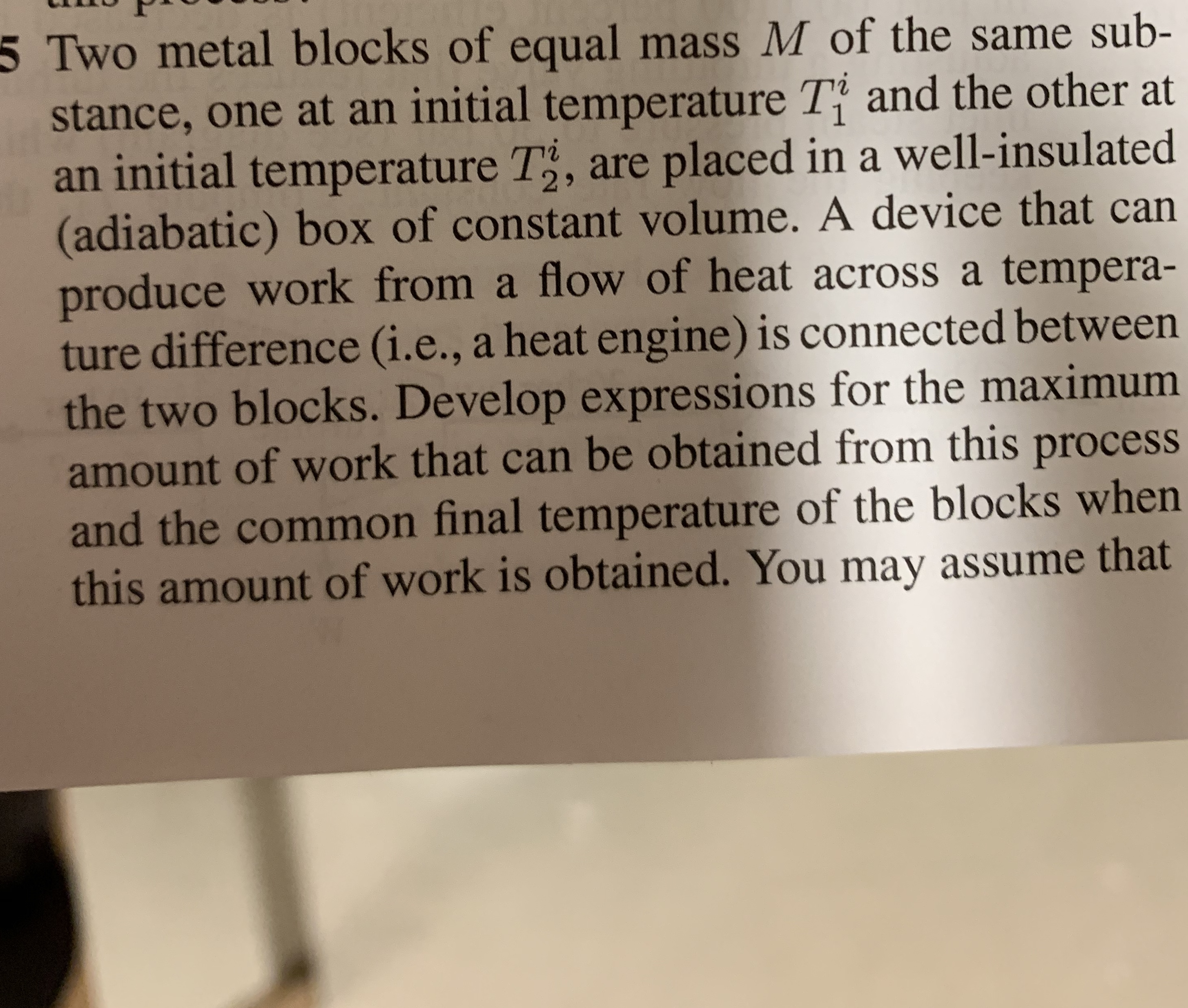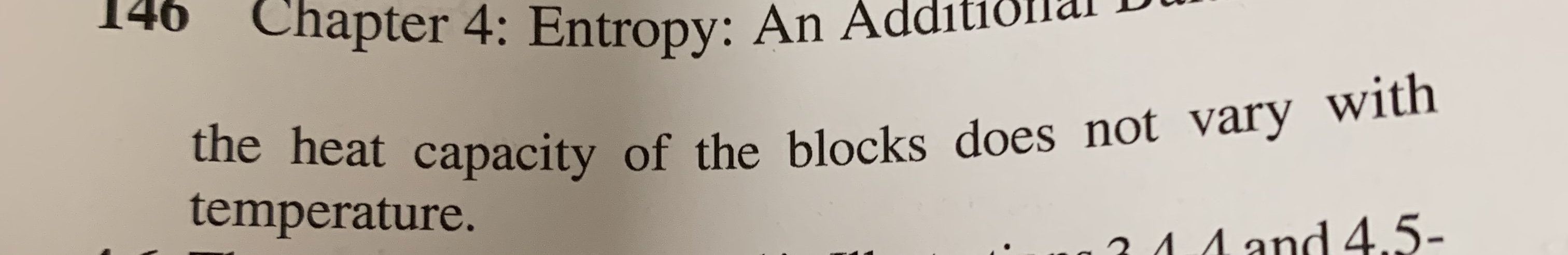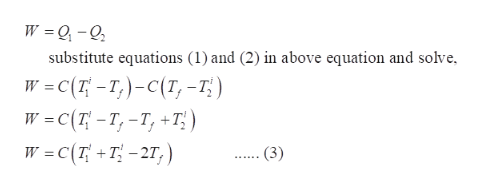# 5 Two metal blocks of equal mass M of the same sub-stance, one at an initial temperature Ti and the other atan initial temperature T2, are placed in a well-insulated(adiabatic) box of constant volume. A device that canproduce work from a flow of heat across a tempera-ture difference (i.., a heat engine) is connected betweenthe two blocks. Develop expressions for the maximumamount of work that can be obtained from this processand the common final temperature of the blocks whenthis amount of work is obtained. You may assume that 14Chapter 4: Entropy: An Additiothe heat capacity of the blocks does not vary withtemperature.24 4 and4.5-

Questionhelp_outlineImage Transcriptionclose5 Two metal blocks of equal mass M of the same sub- stance, one at an initial temperature Ti and the other at an initial temperature T2, are placed in a well-insulated (adiabatic) box of constant volume. A device that can produce work from a flow of heat across a tempera- ture difference (i.., a heat engine) is connected between the two blocks. Develop expressions for the maximum amount of work that can be obtained from this process and the common final temperature of the blocks when this amount of work is obtained. You may assume that fullscreenhelp_outlineImage Transcriptionclose14 Chapter 4: Entropy: An Additio the heat capacity of the blocks does not vary with temperature. 24 4 an d4.5- fullscreen
check_circleExpert Solution
Step 1

In an adiabatic system, the work is delivered by a heat engine (H.E). The temperature of the block with higher temperature will decrease and the temperature of the block with lower temperature will increase until both the blocks attain a final temperature. At this point the heat engine stops operating.

Let the final temperature of both the blocks be Tf. Heat withdrawn from block 1 is Q1  and heat added to block 2 is Q2.

The schematic diagram representing this situation is shown as:

Step 2

Since, the heat capacity of both blocks are assumed to be constant and as both the blocks are of same material, their heat capacity will be same and is denoted by C.

The amount of heat withdrawn from block 1 is calculated as shown by equation (1) and the amount of heat added to the block 2 is calculated as shown by equation (2).

Step 3

The work delivered by the heat ...help_outlineImage TranscriptioncloseW Q-0 substitute equations (1) and (2) in above equation and solve W -C(7-T)-C(T, -T;) W C(T-T,-T, +T) W C(T+T-27,) (3) fullscreen

### Want to see the full answer?

See Solution

#### Want to see this answer and more?

Solutions are written by subject experts who are available 24/7. Questions are typically answered within 1 hour*

See Solution
*Response times may vary by subject and question
Tagged in

### Chemical Engineering